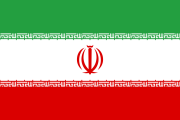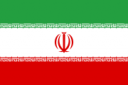# Currency of Iran: Iranian rial, today's rate ﷼The Iranian Rial is the currency of Iran. The code of Iranian rial is IRR. We use as symbol of Iranian rial. The Iranian Rial is divided in 100 dinar. IRR is regulated by The Central Bank of the Islamic Republic of Iran.

## Did you know:

• The most popular conversions of Iranian rial are
• the Iranian rial was introduced in 13 May 1932 (88 years ago).
• There are 7 coins for the Iranian rial ( 50 , 100 , 250 , 500 , 1000 , 2000 and 5000 ),
• the Iranian rial has 10 banknotes ( 100 , 200 , 500 , 1000 , 2000 , 5000 , 10000 , 20000 , 50000 and 100000 )

## Exchange rate of Iranian rial , currency of Iran

### All currencies IRR

currency/IRR Converter
1 INR = 570.5400 IRR
1 USD = 42105.1720 IRR
1 BDT = 496.5718 IRR
1 NOK = 4784.5620 IRR
1 PKR = 263.0746 IRR
1 AFN = 547.1733 IRR
1 EUR = 51044.1000 IRR
1 AED = 11463.3714 IRR
1 AUD = 31262.4636 IRR
1 GBP = 56587.0255 IRR
1 SAR = 11223.8117 IRR
1 NPR = 356.8919 IRR
1 QAR = 11564.1368 IRR
1 NZD = 29673.5244 IRR
1 TRY = 5397.7336 IRR
1 KWD = 138402.1583 IRR
1 RUB = 568.0498 IRR
1 NGN = 110.6570 IRR
1 BTC = 792602863.9485 IRR

## Currency Of Iran